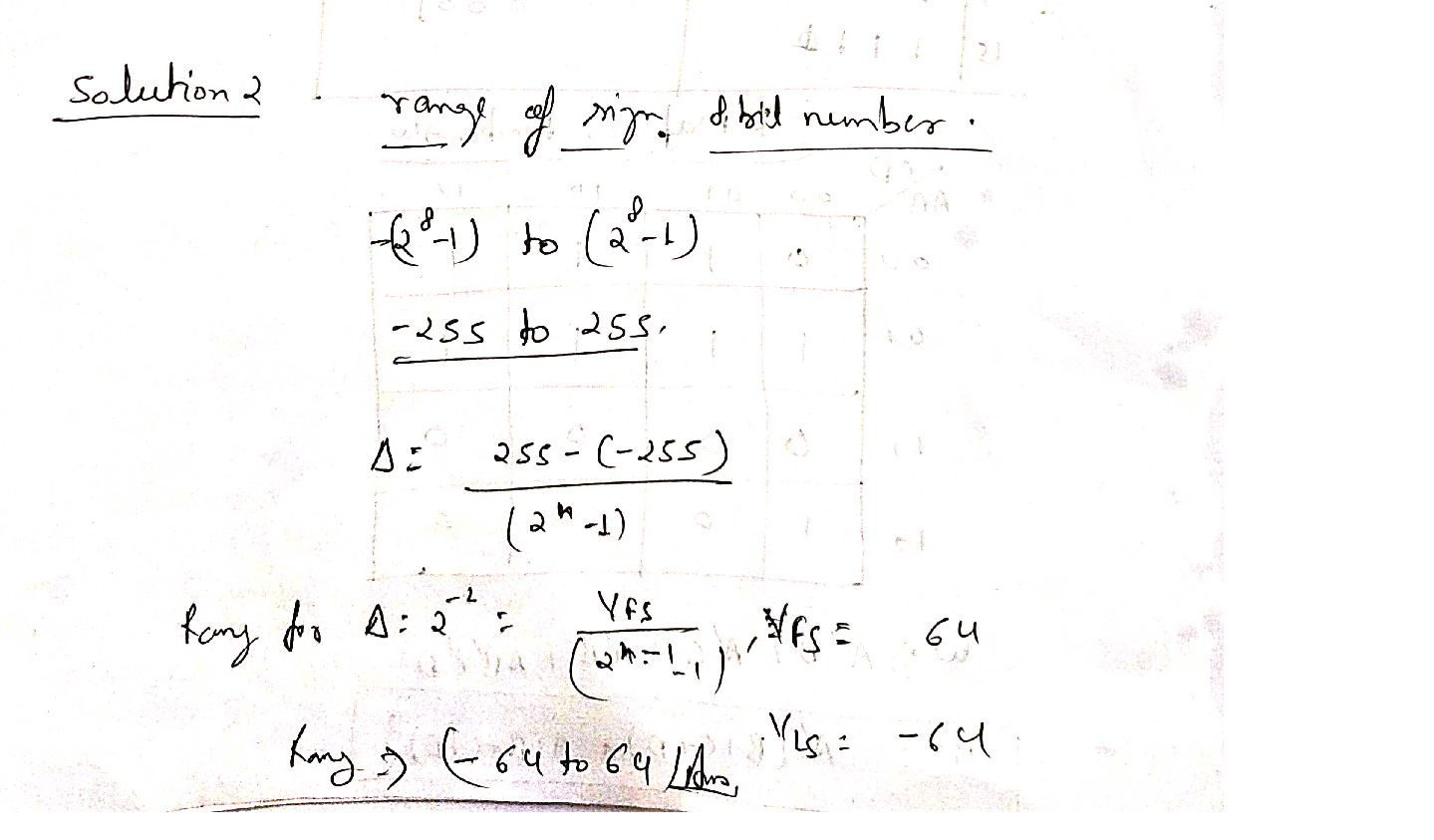Question

Digital Systems

What is the range of a signed 8-bit binary fixed-point system with delta=2²?Verified### Question 40353Digital Systems

Convert -2.71828 to single-precision IEEE 754 floating-point format.

### Question 40352Digital Systems

Suppose the following HEX value represents a single-precision floating number IEEE754 format. Find out the floating-point value it represents.

### Question 40351Digital Systems

Question 8. With a bus clock at 80 MHz, the slowest we can run SysTick interrupts is80,000,000/16777216=4.768 Hz. How could we sample at 1 Hz (trigger ADC exactly every 1sec)?Give a flow chart including a mailbox used to pass data to the foreground.

### Question 40350Digital Systems

Why is binary fixed-point faster than decimal fixed-point?

### Question 40349Digital Systems

What errors occur with these poorly written equations?
I=75-(N / 1024)
\mathbf{I}=\mathbf{N}-(75 / 1024)

### Question 40348Digital Systems

Question 5. What error might occur with multiply? Prove this equation has no overflow(where N comes from a 12-bit ADC)
I=(160-N) / 4096

### Question 40347Digital Systems

Can the sum of two fixed-point numbers overflow? How does one detect overflow?

### Question 40346Digital Systems

Question 3. If range is defined as (max-min) and precision is in bits, what equation related range,precision, and resolution?

### Question 40345Digital Systems

What is the range of a signed 8-bit binary fixed-point system with delta=2²?

### Question 40344Digital Systems

Question 1. What is the range of an unsigned 16-bit decimal fixed-point system with delta=0.01?

### Submit query